## Thursday, 7 August 2014

### chapter-9- Worked Out Examples – 2

 Example: 3
 What is the minimum number of times that a fair coin must be tossed so that the chances of getting at least one Head are greater than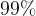$99\%$?
 Solution: 3
In a sequence of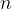$n$ tosses , the probability of obtaining a Tail on every toss is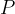$P$(all tails in$n$ tossses)${ =\underbrace{ \dfrac{1}{2} \times \dfrac{1}{2} \times \dfrac{1}{2} \times \;\ldots \times \dfrac{1}{2}}_{\rm{Comment:1}}}$ Comment:1$n$ times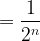$= \dfrac{1}{{{2^n}}}$
because at every toss, the probability of getting a Tail is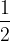$\dfrac{1}{2}$, and also, all tosses are independent of each other.
Thus,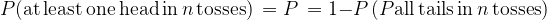$P({\rm{at\, least\, one\, head \,in}}\, n \, {\rm{tosses}}) \, =P\,=1-P\, (P{\rm{all\, tails\, in}}\, n \,{\rm{tosses}}) \,$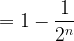$= 1 - \dfrac{1}{{{2^n}}}$
We want$P$ to be greater than$99 \%$, or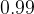$0.99$. Thus,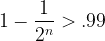$1 - \dfrac{1}{{{2^n}}} > .99$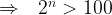$\Rightarrow \,\,\,\, {2^n} > 100$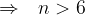$\Rightarrow \,\,\,\, n > 6$
Thus, a minimum of$7$ tosses are required.
 Example: 4
 Two persons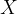$X$ and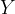$Y$ are playing a game: They throw a coin alternately until one of them gets a Head and wins. How advantageous is it in such a game to make the first throw?
 Solution: 4
Suppose that$X$ makes the first throw. Let us calculate the probability of$X$winning the game.
Let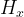${H_x}$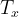${T_x}$ denote a Head and a Tail respectively obtained by$X$. A similar notation follows for$Y$. Now,$X$ will win the game in the following (mutually exclusive) sequences of tosses:
 SEQUENCE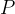$P$ (SEQUENCE)${H_x}$$\dfrac{1}{2}$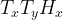${T_x}{T_y}{H_x}$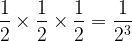$\dfrac{1}{2} \times \dfrac{1}{2} \times \dfrac{1}{2} = \dfrac{1}{{{2^3}}}$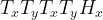${T_x}{T_y}{T_x}{T_y}{H_x}$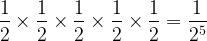$\dfrac{1}{2} \times \dfrac{1}{2} \times \dfrac{1}{2} \times \dfrac{1}{2} \times \dfrac{1}{2} = \dfrac{1}{{{2^5}}}$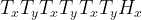${T_x}{T_y}{T_x}{T_y}{T_x}{T_y}{H_x}$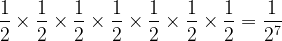$\dfrac{1}{2} \times \dfrac{1}{2} \times \dfrac{1}{2} \times \dfrac{1}{2} \times \dfrac{1}{2} \times \dfrac{1}{2} \times \dfrac{1}{2} = \dfrac{1}{{{2^7}}}$$\vdots$$\vdots$
Thus, the probability of$X$ winning the game is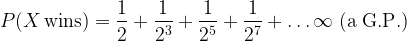$P(X\,{\rm{wins}}) = \dfrac{1}{2} + \dfrac{1}{{{2^3}}} + \dfrac{1}{{{2^5}}} + \dfrac{1}{{{2^7}}} + \ldots \infty \;({\rm{a}}\;{\rm{G}}{\rm{.P}}.)$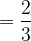$= \dfrac{2}{3}$
This means that one who makes the first throw has twice the chance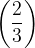$\left( {\dfrac{2}{3}} \right)$of winning than the other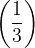$\left( {\dfrac{1}{3}} \right)$